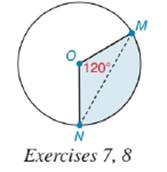Chapter 8.5, Problem 8E### Elementary Geometry for College St...

6th Edition
Daniel C. Alexander + 1 other
ISBN: 9781285195698

#### Solutions

Chapter
Section### Elementary Geometry for College St...

6th Edition
Daniel C. Alexander + 1 other
ISBN: 9781285195698
Textbook Problem
1 views

# If the area of the 1200 sector is 40 cm2 and the area of ∆ M O N is 16 cm2, what is the area of the segment bounded by M N - and M N ^ ?To determine

To find:

Thearea of the segment bounded by MN- and MN^

Explanation

Calculation:

Area of 1200 sector is given as 40 cm2.

Area of MON is given as 16 cm2.

From the diagram, it is clear that:

Area of 1200 sector = Area of MON+ Area of the segment bounded by MN- and MN^

Area of the segment bounded by MN- and MN^ = Area of 1200 sector - Area of MON

Let’s substitute the values of area of sector and MON in the above expression to find the area of

### Still sussing out bartleby?

Check out a sample textbook solution.

See a sample solution

#### The Solution to Your Study Problems

Bartleby provides explanations to thousands of textbook problems written by our experts, many with advanced degrees!

Get Started

#### Find more solutions based on key concepts